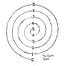Restating my previous phrasing:"Gate of Five Angles" is thus Gate-190, the total sum of all natural numbers from 1 to 19.1+9 = 10Expand full commentYou really like doing this, don't you? ;)"FIVE ANGLES" = AQ-190→ 190 (base-10) = 5A (base-36)So... 5 Angles."SUMERIAN IDEAL YEAR" = AQ-333→ Actually you missed by 27 =)"QABBALISTIC ODDMENTS" = AQ-360→ Now you did it! Hahaha.Expand full commentMore to come, but here's a few notes building on §04 to start people off toying with this.(Brief explanatory opener — AQ values have, bracketed, single-lemur derivations (utilizing a tangential method to the later application of the hex cycle — accessible (without documentation) in the xeno.cx gematrix (briefly stated (each step continuously applied, and prioritized in order): sum pairs — 3=147; 6=285 — sum same-cycle numbers)), and decimal reduction of the AQ.)Decimal numerals listed with values in primitive method & AQ:ZERO (₱ 4) = AQ 100 (= 10 = 1)ONE (₱ 3) = AQ 61 (= 7)TWO (₱ 3) = AQ 85 (= 4)THREE (₱ 5) = AQ 101 (= 20 = 2)FOUR (₱ 4) = AQ 96 (= 6)FIVE (₱ 4) = AQ 78 (= 87 = 6)SIX (₱ 3) = AQ 79 (= 97 = 7)SEVEN (₱ 5) = AQ 110 (= 20 = 2)EIGHT (₱ 5) = AQ 94 (= 4)NINE (₱ 4) = AQ 78 (= 87 = 6)Instructions from §04 followed, including supplementary information from above, along with sums of both ₱ and AQ values for a given syzygy:ZERO (₱ 4) (AQ 100 (= 10 = 1)) + NINE (₱ 4) (AQ 78 (= 87 = 6)) = (₱ 8) (AQ 178 (= 70 = 7)).ONE (₱ 3) (AQ 61 (=7)) + EIGHT (₱ 5) (AQ 94 (= 4)) = (₱ 8) (AQ 155 (= 20 = 2)).TWO (₱ 3) (AQ 85 (= 4)) + SEVEN (₱ 5) (AQ 110 (= 20 = 2)) = (₱ 8) (AQ 195 (= 51 = 6)).THREE (₱ 5) (AQ 101 (= 20 = 2)) + SIX (₱ 3) (AQ 79 (= 97 = 7) = (₱ 8) (AQ 180 (= 81 = 9)).FOUR (₱ 4) (AQ 96 (= 6)) + FIVE (₱ 4) (AQ 78 (= 87 = 6)) = (₱ 8) (AQ 174 (= 30 = 3)).A "modest" AQ-driven case for a different ordering, that constructs further pairings:SIX + THREE (= AQ 180 (= 81 = 9)).TWO + SEVEN (= AQ 195 (= 51 = 6)).FIVE + FOUR (= AQ 174 (= 30 = 3)).(195 + 174 = 369 (= 63 = 9)) (51 + 30 = 81 = 9) (6 + 3 = 9).ONE + EIGHT (AQ 155 (= 20 = 2)).NINE + ZERO (AQ 178 (= 70 = 7)).(155 + 178 = 333 (= 63 = 9)) (20 + 70 = 90 = 9) (2 + 7 = 9).Expand full comment